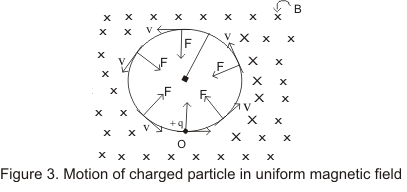# Lorentz Force |Motion of a charged particle in magnetic field

## (3) Lorentz Force

• We know that force acting on any charge of magnitude q moving with velocity v inside the magnetic field B is given by
F=q(v X B)
and this is the magnetic force on charge q due to its motion inside magnetic field.
• If both electric field E and magnetic field B are present i.e., when a charged particle moves through a reagion of space where both electric field and magnetic field are present both field exert a force on the particle and the total force on the particle is equal to the vector sum of the electric field and magnetic field force.
F=qE+q(v X B)                   (4)
• This force in equation(4) is known as Lorentz Force.
• Where important point to note is that magnetic field is not doing any work on the charged particle as it always act in perpendicular direction to te motion of the charge.

## (4)Motion of Charged Particle in The Magnetic Field

• As we have mentioned earlier magnetic force F=(vXB) does not do any work on the particle as it is perpendicular to the velocity.
• Hence magnetic force does not cause any change in kinetic energy or speed of the particle.
• Let us consider there is a uniform magnetic field B perpendicular to the plane of paper and directed in downward direction and is indicated by the symbol C in figure shown below.• Now a charge particle +q is projected with a velocity v to the magnetic field at point O with velocity v directed perpendicular to the magnetic field.
• Magnetic force acting on the particle is
F=q(v X B) = qvBsinθ
Since v is perpendicular to B i.e., angle between v and B is θ=90 Thus charged particle at point O is acted upon by the force of magnitude
F=qvB
and the direction of force would be perpendicular to both v and B
• Since the force f is perpendicular to the velocity, it would not change the magnitude of the velocity and the peffect of this force is only to change the direction of the velocity.
• Thus under the action of the magnetic force of the particle will more along the circle perpendicular to the field.
• Therefore the charged particle describe an anticlockwise circular path with constant speed v and here magnetic force work as centripetal force. Thus
F=qvB=mv2/r
where radius of the circular path traversed by the particle in the magnetic in field B is given as
r=mv/qB                   ---(5)
thus radius of the path is proportional to the momentum mv pof the charged particle.
• 2πr is the distance traveled by the particle in one revolution and the period T of the complete revolution is
T=2 πr /v
From equation(5)
r/v=m/qB
time period T is
T=2πm/qB                   (6)
and the frequency of the particle is f=1/T=qB/2πm                   (7)
• From equation (6) and (7) we see that both time period and frequency does not dependent on the velocity of the moving charged particle.
• Increasing the speed of the charged particle would result in the increace in the radius of the circle. So that time taken to complete one revolution would remains same.
• If the moving charged particle exerts the magnetic field in such a that velocity v of particle makes an angle θ with the magnetic field then we can resolve the velocity in two components

vparallel : Compenents of the velocity parallel to field
vperpendicular :component of velocity perpendicular to magnetic field B
• The component vpar would remain unchanged as magnetic force is perpendicular to it.
• In the plane perpendicular to the field the particle travels in a helical path. Radius of the circular path of the helex is

r=mvperpendicular/qB=mvsinθ/qB                   (8)

Note to our visitors :-

Thanks for visiting our website.